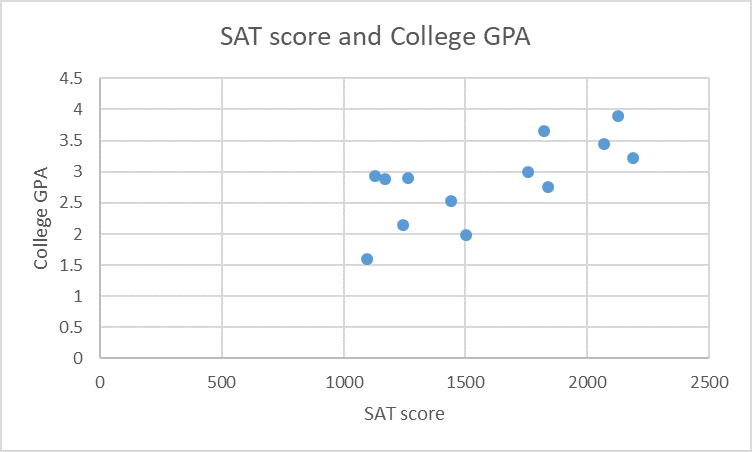# SAT score and College GPA4.543.532.521.510.505001000150020002500SAT scoreCollege GPAst

Question
1 views

Using the chart as a guide, what value of the linear correlation coefficient (r) makes sense according to how the data is trending?

SAT score_GPA scatterplot.pdfhelp_outlineImage TranscriptioncloseSAT score and College GPA 4.5 4 3.5 3 2.5 2 1.5 1 0.5 0 500 1000 1500 2000 2500 SAT score College GPA st fullscreen
check_circle

Step 1

The scatterplot and the corresponding correlation coefficient is given below:

If there is perfect negative relationship between two variables, then the value of correlation coefficient is approximately ­–1. this is shown below:

Step 2

If there is perfect positive relationship between two variables, then the value of correlation coefficient is approximately 1. this is shown below:

Step 3

If there is moderate negative relationship between two variables, then the value of c...

### Want to see the full answer?

See Solution

#### Want to see this answer and more?

Solutions are written by subject experts who are available 24/7. Questions are typically answered within 1 hour.*

See Solution
*Response times may vary by subject and question.
Tagged in

### Data